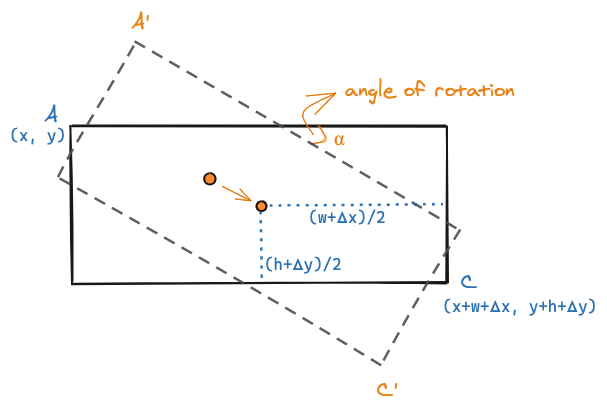# Resizing Rotated Elements

Visual editors usually allow resizing and rotation of elements. Applying both transforms can be a little tricky. This post explores an algorithm to implement this feature.

## Introduction

If you have ever used a visual editor — a WYSIWYG designer or a Graphics editor, you'd expect to be able to resize and rotate the selected shape or element. These are common operations, and yet when applied together can cause a bit of an itchy head.

## The Problem

Let's take the most common use case - a rectangle (Elements on a web page have a bounding rectangle, and vectors in graphics have a rectangular bounding box). We usually represent the rectangle with four numbers - the `x, y` coordinates of the top-left corner of the rectangle, and the `width, height` of the rectangle.

For resizing this rectangle, common practice is to add four draggable handles at the corners of the rectangle. You can also add four additional handles in the middle of each side to resize in only one direction. For simplicity, I'm going to just add one handle - handle to move the bottom right corner of the rectangle.

To resize, one calculates how much `x` and `y` units the user has dragged the handle. Let's call the change in the values to be `𝝙x` and `𝝙y`. The new width and height of the rectangle would be `width + 𝝙x` and `height + 𝝙y` respectively.

Try dragging the bottom right handle in the rectangle below to see it resize.

In the interactive example above, there'a top handle which can be used to rotate the rectangle. Try rotating the rectangle above and then resize it. What do you see?

You will notice a couple of issues:

1. When you resize the rectangle, the shape tends to move. i.e. you are dragging the bottom-right corner, but somehow the top-left corner is also moving.
2. The second one may be a bit more subtle to notice. The distance you drag horizontally or vertically does not quite match with the change in size you perceive in the rotated shape.

## What's Happening?

The rectangle is rotated about its center. In the diagram below `A'` is the new location of the top-left corner `A`.

When you increase the width by `𝝙x` and height by `𝝙x` you end up moving the center of the shape. Even though you have not changed the coordinates of `A`, the coordinates of `A'` will be different.

This accounts for the first issue. The second issue is that we are changing the `width` and `height` based on the `x` and `y` changes of the bottom-right corner. That would be totally fine when the shape has not been rotated. But for rotated shapes, one needs to calculate the width and height changes based on the angle of rotation. The diagram below represents these changes as `𝝙x'` and `𝝙y'`.

## The Solution - Do not move A'

Let's address the first issue. As we move the bottom-right corner we want to ensure that the top-left corner does not change. Which means, when we resize a rotated rectangle, we should also update its position.

Let's calculate the coordinates for `A'`. We can use a rotation matrix to do that. We also have to consider that the rotation does not happen around the origin of the canvas, but around the center of the rectangle.

First let's establish the center of the rectangle `cx, cy`:

``// For a rectangle with top-left at x, yconst cx = rectangle.x + rectangle.width / 2;const cy = rectangle.y + rectangle.height / 2;``

Now applying the combined matrix, we can create a `rotate` function that returns the coordinates after rotation:

``function rotate(x, y, cx, cy, angle) {  return [    (x - cx) * Math.cos(angle) - (y - cy) * Math.sin(angle) + cx,    (x - cx) * Math.sin(angle) + (y - cy) * Math.cos(angle) + cy,  ];}const rotatedA = rotate(rectangle.x, rectangle.y, cx, cy); // calculate A'``

As we were dragging the bottom-right corner of the rectangle, we knew what the value of `C'` (`rotatedC` in the code) should be. Since we want `A'` to remain the same, we can now calculate the new center by finding the midpoint between `A'` and `C'`.

``const newCenter = [  (rotatedA + rotatedC) / 2,  (rotatedA + rotatedC) / 2,];``

Now to calculate the new top-left coordinates for the rectangle, we simply rotate `A'` around the new center by the reverse angle `-angle`.

``const newA = rotate(rotatedA, rotatedA, newCenter, newCenter, -angle);``

Setting the `x, y` of the rectangle to `newA` will ensure that the rotated rectangle does not visually shift when resized.

Now let's deal with the second issue - we need a projection of what the width and height should be when there is no rotation. The answer is to build on top of the first solution.

We can calculate the unrotated coordinates of the bottom-right corner `C` by rotating the new coordinates of `C'` around the new center by the reverse angle.

``const newC = rotate(rotatedC, rotatedC, newCenter, newCenter, -angle);``

The width and height of the rectangle can be calculated by measuring the difference in `x` and `y` values of `newC` and `newA`.

``const newWidth = newC - newA;const newHeight = newC - newA;``

## Putting it all together

Here's the solution implemented for you to play with:

The code put together:

``function adjustRectangle(rectangle, bottomRightX, bottomRightY, angle) {  const center = [    rectangle.x + rectangle.width / 2,    rectangle.y + rectangle.height / 2  ];  const rotatedA = rotate(rectangle.x, rectangle.y, cx, cy);  const newCenter = [    (rotatedA + bottomRightX) / 2,    (rotatedA + bottomRightY) / 2,  ];  const newTopLeft = rotate(    rotatedA,    rotatedA,    newCenter,    newCenter,    -angle  );  const newBottomRight = rotate(    bottomRightX,    bottomRightY,    newCenter,    newCenter,    -angle  );  rectangle.x = newTopLeft;  rectangle.y = newTopLeft;  rectangle.width = newBottomRight - newTopLeft;  rectangle.height = newBottomRight - newTopLeft;}``